# How to Calculate Standard Deviation in Excel [STDEV.S Formula]

Posted May 21, 2019 by Sheraz Ali in Microsoft ExcelIf you’re someone who has to deal with a lot of numbers on a daily basis, you must familiarize yourself with a large array of Excel formulae. To perform basic mathematical operations, such as addition, multiplication, subtraction and Standard deviation (SD), you must learn how these formulae work.

I’ll teach you how to calculate Sample Standard deviation and Standard Deviation for a population. But first, let’s get friendly with the basics.

### What is Standard Deviation and Why Calculate it?

Standard deviation is used to tell how far a value is spread from the mean value of a group or a population.

If you get a high value of SD, it means the numbers vary a lot from the average or expected value. If the SD is on the low side, your individual numbers are close to the average or mean.

In eighth or ninth grade, to calculate SD you had to go through a complicated series of steps. Excel has made life a hell lot easier. All you have to do now is insert the SD population and Sample formulae in Excel to get the intended results.

### Difference Between Sample and Population Standard Deviation

There are certain differences between calculating SD in entire populations and limited groups or samples in Excel.

Qualitatively, The SD of a population is fixed and yielded from every unit of a said population. Sample SDs are targeted on a limited number of individuals or units of a population.

They have a greater chance of variability and are manifolds greater than Population SDs.

In Excel terms, Population Standard Deviation takes into account all data points ( N). Sample Standard Deviation (SSD), on the other hand, takes into account all data points minus one value (N-1).

### How to Calculate Standard Deviation in Excel (Sample)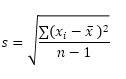1. Open the Microsoft Excel Document containing your data.2. Click an empty cell. Any will suffice.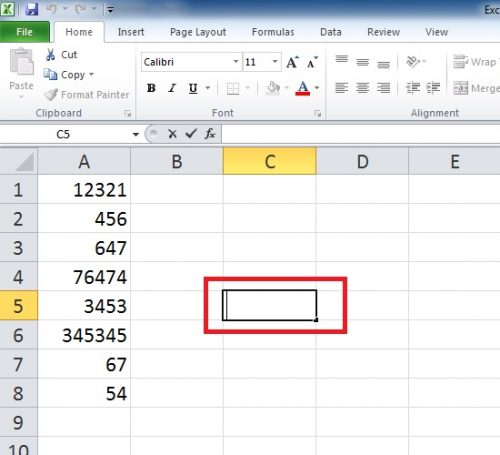3. Start your Standard Deviation formula with =.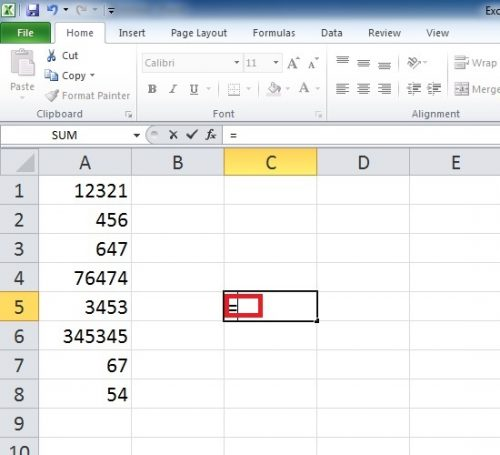4. Type STDEV.S.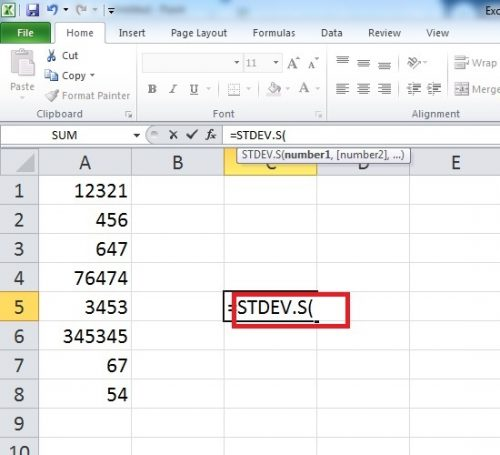5. Select the cells you want the Standard Deviation computed for.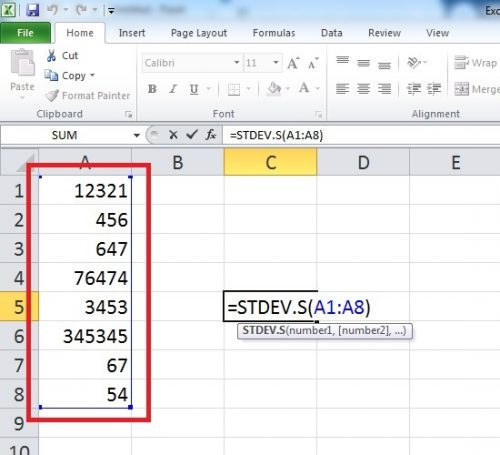• You can select the entire data or scattered cell references ( A1, A4 etc.) depending upon your preferences.

6. Press Enter.

7. Your Standard deviation is here.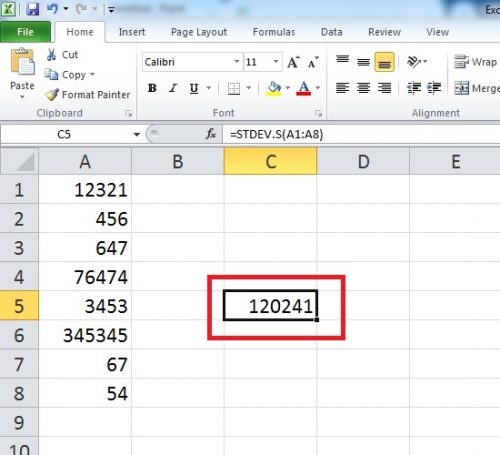### How to Calculate Standard Deviation in Excel ( Population)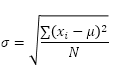To calculate SSP or Standard Deviation Population, follow the aforementioned steps with a minor modification.

Instead of STDEV.S, Insert the Formula STDEV.P and you’re good to go.

Read More: How to Divide in Excel : Division Formulae

### Note: Standard Deviation of ZERO

If the numbers are the same, there’s no need to calculate SD. Same numbers means zero variability which in turn means SD=0.

For example, if all the numbers on my Excel sheet are 10, then the SD will be equal to 0.

Still not sure how to calculate Standard Deviation in Excel? Leave a comment below and we will get back to you. Additional, check this youtube video tutorial for further guidance.